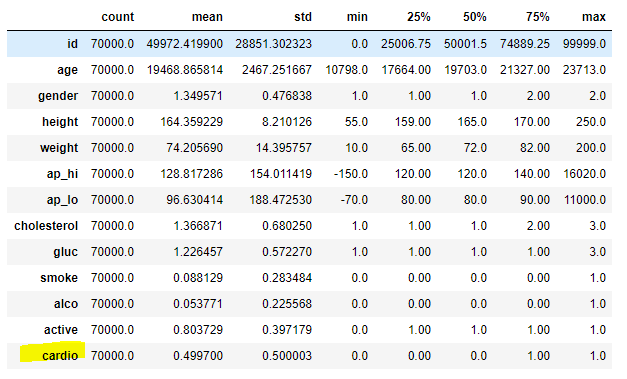Call Us @

(91) 96200 48623

## Blog: Deep Neural Network for Structured Data - Heart Attack Prediction From ScratchDeep Neural Network for Structured Data - Heart Attack Prediction From Scratch

Preventive and predictive methods can help in managing the devastating effect of heart diseases. In this blog, we aim to show simple steps involved in building a predictive model using the Deep Neural Network method to predict a heart attack.

A detailed overview of Heart Attack Prediction has been discussed here

In this tutorial, we are going to use the heart attack prediction dataset available on Kaggle.

In this heart attack prediction dataset, structured information - factual (e.g. age, height, gender, weight, etc), medical examination results (e.g. BP, Glucose, etc), and behavioral/subjective given by patient (e.g. smoking, taking alcohol, level of physical activity, etc) - is available.

```import pandas as pd cardio = pd.read_csv("cardio_train.csv", sep=";")```

```# Summary Statistics cardio.describe().transpose()```### Feature Engineering - Stage 1

A detailed exploratory data analysis has been done to understand data, find the distribution of each of the independent variables/ features, and perform bivariate analysis - relationship between label variable and each of the independent variables/features.

Here are some of the data treatments done and also the features to be created. After that, we create an additional list of features.

```import numpy as np # Age in Years cardio['age_years'] = round(cardio['age']/365,0) # Outlier Treatment: Height cardio['height'] = np.where(cardio['height']>207,207,cardio['height'])```

```# Pressure - High: Category def ap_hi (values):     if values<=120:         return 1     elif 120<values<=200:         return 2     else:         return 3 cardio['ap_hi_cat']=cardio.ap_hi.apply(lambda x: ap_hi(x) ) # Outlier Treatment: ap_hi cardio['ap_hi'] = np.where(cardio['ap_hi']>200,201,cardio['ap_hi'])```

```# Pressure - Low: Category def ap_lo (values):     if values<=50:         return 1     elif 50<values<=120:         return 2     else:         return 3     cardio['ap_lo_cat']=cardio.ap_lo.apply(lambda x: ap_lo(x) )```

```# Capping def capping(series, lowMax, highMin):     if series <lowMax:         return lowMax     elif series>highMin:         return highMin     else:         return series      cardio['ap_hi'] = cardio.ap_hi.apply(lambda x: capping(x,50,120) ) cardio['ap_lo'] = cardio.ap_lo.apply(lambda x: capping(x,50,120) )  # Scale up```

```# Pressure - Low: modulus cardio['ap_lo_mod_10'] = np.where(cardio['ap_lo']%10==0,1,0)```

```# BMI Cal cardio['bmi'] = np.round(cardio['weight']/((cardio['height']/100)*(cardio['height']/100)),0)```

```# Outlier Treatment: BMI import numpy as np cardio['bmi'] = np.where(cardio['bmi']>50,50,cardio['bmi'])```

```# Create multiple group using lamda function def bmicat(values):     if values <=18.5:         return 1     elif 18.5<values<=24.9:         return 2     elif 24.9<values<=29.9:         return 3     else:         return 4      # BMI - Categories cardio['bmi_cat'] = cardio.bmi.apply(lambda x: bmicat(x) ) ```

```# Pressures - difference and ratio cardio['s_d_ratio'] = np.round(np.abs(np.min(cardio['ap_hi']))+1+cardio['ap_hi'],2)/(np.abs(np.min(cardio['ap_lo']))+1+cardio['ap_lo']) cardio['s_d_diff'] = np.round(cardio['ap_hi']-cardio['ap_lo'],2)```

```# Capping Ratio and Difference cardio['s_d_ratio'] = cardio.s_d_ratio.apply(lambda x: capping(x,1.2,1.5) ) cardio['s_d_diff'] = cardio.s_d_diff.apply(lambda x: capping(x,0,50) ) ```

```# EDA - Bivariate Analysis: Dummy Variables cardio['age_above_55'] = np.where(cardio['age_years']>55,1,0) cardio['s_d_diff_above_45'] = np.where(cardio['s_d_diff']>45,1,0) cardio['ap_lo_above_85'] = np.where(cardio['ap_lo']>85,1,0) cardio['ap_hi_above_125'] = np.where(cardio['ap_hi']>125,1,0)```

### Tags

• SOCIAL SHARE :